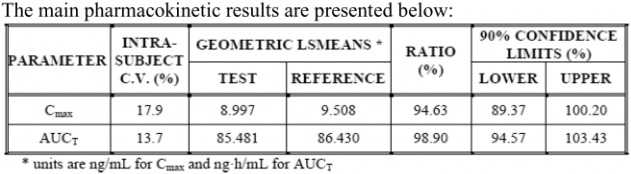## Manipulations with PK data in BE analysis [General Sta­tis­tics]

Dear Elena,

I am not a statistician, but I should be able to answer in lay language» Specifically, I would like to know when we should perform ln transformation: we calculate Geom mean and then transform its value to ln value and do ANOVA or we transform each concentration Cmax to Ln-data and then calculate geom mean.

Log-transform each value and then calculate the arithmetic (not geometric) mean of the log-transformed values. If you calculate the exponential of this arithmetic mean, it will give you the geometric mean on the original scale.

» I also would like to know how GMR is usually presented in tables. Whether it is usually GMR of ln-transformed or non-transformed data? I provide the table below as an example and would like to know the origin of its values as I asked above.What you have in your table is the non-transformed data. Just try and calculate the exponential: the values you would get for AUC would be really extreme. If reporting log-transformed data: you would need to calculate a difference, not a ratio.

The ratio in your table is not a geometric mean ratio and is therefore rightly not labelled GMR. It is a ratio of LSMEAN, not of geometric means. It makes no difference if your study is balanced (i.e. same number of subjects with sequence TR and sequence RT, in which case the LSMEAN is equal to the geometric mean), but if you have a drop-out and your study gets unbalanced you will get some differences.

Regards
OhlbeIng. Helmut Schütz